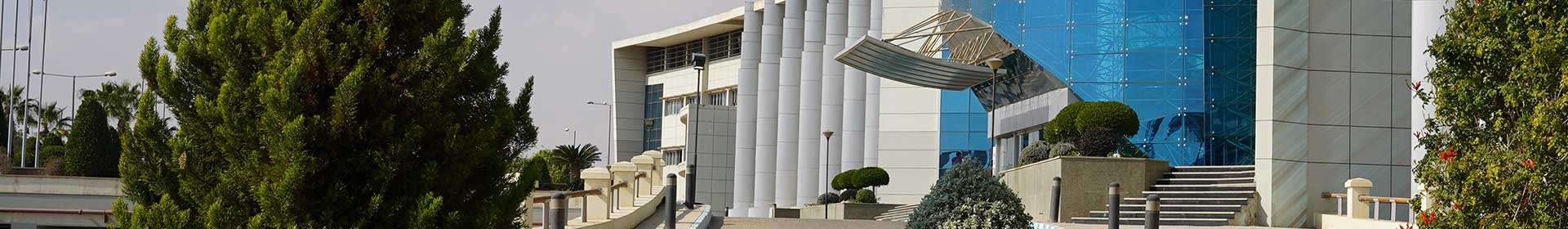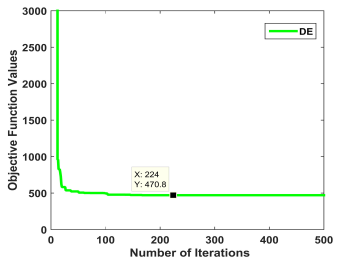Conference Paper

# Solution of Uncertain Solid Transportation Problem by Integer Gaining Sharing Knowledge Based Optimization Algorithm

By
Agrawal P.
Ganesh T.
Mohamed A.W.

This paper presents the application of gaining sharing knowledge (GSK) based optimization algorithm to an uncertain solid transportation (UST) problem. The UST problem consists of supply, demand, and conveyance constraints under uncertain environment. To solve the said problem, the expected criterion model is considered so that the expected value of the objective function is minimized. 99-method generates the expected value of the assumed uncertain variables, and the transformed problem is solved. Due to the consideration of integer decision variables, GSK is modified to integer gaining sharing knowledge based optimization algorithm (IGSK). IGSK, along with 99-method i.e., hybrid algorithm solves the considered problem. A numerical example illustrates the methodology, and the obtained results are compared with other metaheuristic algorithms and the global optimal solution. It indicates that the IGSK performs same as other metaheuristic algorithms in terms of convergence, ability of finding optimal solution, and robustness. © 2020 IEEE.